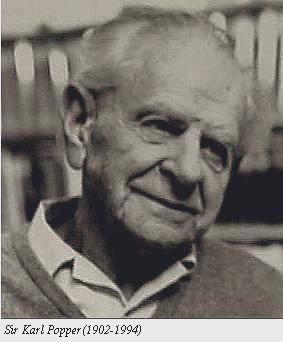# The Box-Muller transformation

Let$N_1,N_2$ be independent$N(0,1)$ random variates.$(N_1,N_2)$ defines a point in Cartesian coordinates. We transform to polar by$N_1 = R \cos \theta$$N_2 = R \sin \theta$

This transformation is one to one and has continuous derivatives. So we can derive the joint distribution$f_{R,\theta}(r,\theta)$ by using$f_{R,\theta}(r,\theta) = f_{N_1,N_2}(n_1,n_2)|J^{-1}|$

where$J$ is the Jacobian of the transformation and$J^{-1}(r,\theta )= {\begin{bmatrix}{\dfrac {\partial n_1}{\partial r}}{\dfrac {\partial n_1}{\partial \theta }} \\ {\dfrac {\partial n_2}{\partial r}}{\dfrac {\partial n_2}{\partial \theta }} \end{bmatrix}}$   =$\begin{bmatrix} \cos \theta & -r\sin \theta \\ \sin \theta & r\cos \theta \end{bmatrix}$

Hence$|J^{-1}| = r \cos^2 \theta + r \sin^2 \theta = r$

and$f_{R, \theta} (r, \theta) = r \frac{1}{2\pi} e^{- \frac{1}{2}(n_1^2+n_2^2)} = \boxed{\frac{r}{2 \pi}e^{-\frac{1}{2}(r^2)}}, 0 \leq \theta \leq 2 \pi, 0 \leq r \leq \infty$

And we see$\theta \sim unif[0,2\pi]$ (since$f_{\Theta}(\theta) = \frac{1}{2\pi}$ ) and$R^2 \sim \exp(1/2)$

Hence, to simulate$\Theta$ we simply take$2 \pi U_2$ where$U_2 \sim unif[0,1]$

and to simulate$R$ we can take$-2\ln U_1$ where$U_1 \sim unif[0,1]$.

( to see why find the density of$X=-\ln U, U \sim unif[0,1]$).
So, Box and Muller simply inverted$N_1= R \cos \theta$,$N_2= R \sin \theta$ and moved from$(R,\Theta)$ to$(N_1,N_2)$ by simulating$\Theta$ from$2 \pi U_2$, and an independent$R$ from$\sqrt{- 2 \ln U_1}$

# Hoeffding’s Inequality

Let${X_1,...,X_n}$ be independent zero-mean real valued variables and let${S_n= \sum\limits_{i=1}^{n} X_i.}$ Then$\displaystyle \begin{array}{rcl} \text{If } a_i \leq X_i \leq b_i; \qquad &i&=1,..,n \text{ where } a_1, b_1,...,a_n, b_n \text{ constant then } \\ P(|S_n| \geq t) &\leq& 2 \exp \left( - \frac{2t^2}{\sum\limits_{i=1}^{n} (b_i-a_i)^2} \right), \qquad t>0 \end{array}$

# Bayesian, frequentist, and Fisherian influences, on 15 major statistical topics, 1950s through 1990s# Almost sure convergence via pairwise independence

If${A_1,A_2,...}$ are pairwise independent and${\sum_{n=1}^{\infty}P(A_n)=\infty}$ then as${n \rightarrow \infty}$$\displaystyle \boxed{ \frac{\sum_{m=1}^{n}\mathbb{I}_{A_m}}{\sum_{m=1}^{n}P(A_m)} \xrightarrow{a.s.} 1 }$

Proof:

Let${X_m = \mathbb{I}_{A_m}}$ and${S_n = X_1+...+Xn}$. Since${A_m}$ are pairwise independent, the${X_m}$ are uncorrelated and thus$\displaystyle var(S_n) = var(X_1) + ... + var(X_n)$

Since${X_m \in \{0,1 \}}$$\displaystyle var(X_m) \leq \mathbb{E}[X_m^2] = \mathbb{E}[X_m] \Rightarrow var(S_n) \leq \mathbb{E} [S_n]$

# An inequality of the mean involving truncation

Let${X_1,X_2,...}$ be i.i.d. r.vs with${\mathbb{E}[|X_i|] < \infty}$ and${Y_k = X_k \mathbb{I}_{(|X_k| \leq k)}}$. Then$\displaystyle \boxed{ \mathbb{E}[X_1] \geq \sum_{k=1}^{\infty} \frac{var(Y_k)}{4 k^2 } }$

Proof:

First we proove the following useful result

If${X \geq 0}$ and${ a > 0}$ then$\displaystyle \boxed{ \mathbb{E}[X^a] = \int_{0}^{\infty} a x^{a-1} P(X >x) dx }$$\displaystyle \begin{array}{rcl} \int_{0}^{\infty} a x^{a-1} P(X >x) dx &=& \int_{0}^{\infty} \int_{\Omega}a x^{a-1} \mathbb{I}_{(X>x)} dP dx \\ &=& \int_{\Omega} \int_{0}^{\infty} a x^{a-1} \mathbb{I}_{(X>x)} dP dx \\ &=& \int_{\Omega} \int_{0}^{X} a x^{a-1} dP dx = \mathbb{E}[X^a] \end{array}$

Note you can find the same lemma on Feller Vol.2 (p. 150) as$\displaystyle \mathbb{E}[X^a]= \int_{0}^{\infty} x^a F \{ dx \} = a \int_{0}^{\infty} x^{a-1} [ 1- F(x)] dx$

# Karl Popper: Conjectures and Refutations(1) It is easy to obtain confirmations, or verifications, for nearly every theory-if we look for confirmations.

(2) Confirmations should count only if they are the result of risky predictions; that is to say, if, unenlightened by the theory in question, we should have expected an event which was incompatible with the theory–an event which would have refuted the theory.

(3) Every ‘good’ scientific theory is a prohibition: it forbids certain things to happen. The more a theory forbids, the better it is.

(4) A theory which is not refutable by any conceivable event is nonscientific. Irrefutability is not a virtue of a theory (as people often think) but a vice.

(5) Every genuine test of a theory is an attempt to falsify it, or to refute it. Testability is falsifiability; but there are degrees of testability: some theories are more testable, more exposed to refutation, than others; they take, as it were, greater risks.

(6) Confirming evidence should not count except when it is the result of a genuine test of the theory; and this means that it can be presented as a serious but unsuccessful attempt to falsify the theory. (I now speak in such cases of ‘corroborating evidence’.)

(7) Some genuinely testable theories, when found to be false, are still upheld by their admirers–for example by introducing ad hoc some auxiliary assumption, or by re-interpreting theory ad hoc in such a way that it escapes refutation. Such a procedure is always possible, it rescues the theory from refutation only at the price of destroying, or at least lowering, scientific status.

—————————-

Excerpt from a lecture given by Karl Popper at Peterhouse, Cambridge, in Summer 1953, as part of a course on Developments and trends in contemporary British philosophy.

# Some notes on Kalman Filtering

State Space form

Measurement Equation$\displaystyle \boxed{\mathbf{\underbrace{y_{t}}_{N \times 1}=\underbrace{Z_{t}}_{N \times m}\underbrace{a_{t}}_{m \times 1}+d_{t}+\varepsilon_{t}}}$$\displaystyle Var(\varepsilon_{t})= \mathbf{H_{t}}$

Transition Equation$\displaystyle \boxed{\mathbf{\underbrace{a_{t}}_{m \times 1} =\underbrace{T_{t}}_{m \times m} a_{t-1}+c_{t}+\underbrace{R_{t}}_{m \times g} \underbrace{\eta_{t}}_{g \times 1}}}$$\displaystyle Var(\eta_{t})=\mathbf{Q}_{t}$$\displaystyle E(a_{0})= \mathbf{a_{0} \; \; \; \; Var(a_{0})=P_{0}} \; \; \; \; E(\varepsilon_{t}a_{0}^{\top}) \; \; \; E(\eta_{t}a_{0}^{\top})$

Future form$\displaystyle \mathbf{a_{t+1}=T_{t}a_{t}+c_{t}+R_{t}\eta_{t}}$

# Expectation: Useful properties and inequalitiesIf${X \geq 0}$ is a random variable on${(\Omega, \mathcal{F}, P)}$. The expected value of${X }$ is defined as$\displaystyle \mathbb{E}(X) \equiv \int_{\Omega} X dP = \int_{\Omega} X(\omega) P (d \omega)$

Inequalities

• Jensen’s inequality. If${\varphi}$ is convex and${E|X|, E|\varphi(X)| < \infty}$$\displaystyle \mathbb{E} (\varphi(X)) \geq \varphi(\mathbb{E}X)$

• Holder’s inequality. If${p,q \in [1, \infty]}$ with${1/p + 1/q =1}$ then$\displaystyle \mathbb{E}|XY| \leq \|X\|_p \|Y\|_q$

• Cauchy-Schwarz Inequality: For${p=q=2}$$\displaystyle \mathbb{E}|XY| \leq \left( \mathbb{E}(X^2) \mathbb{E}(Y^2) \right)^{1/2}$

# A nice chart of univariate distribution relationships# Big Data for Volatility vs.Trend

So different aspects of Big Data — in this case dense vs. tall — are of different value for different things.  Dense data promote accurate volatility estimation, and tall data promote accurate trend estimation.

More (No Hesitations blog)